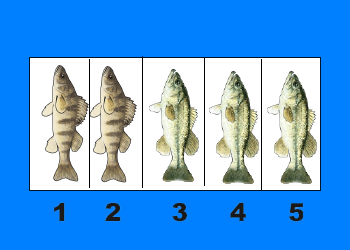# comparing fractionsEquivalent fractions are different fractions that name the same number. The numerator and the denominator of a fraction must be multiplied by the same nonzero whole number in order to have equivalent fractions. The presentations and videos below provide multiple explanations and examples. Using Equivalent Fractions on a Number Line I really like this PPT […]